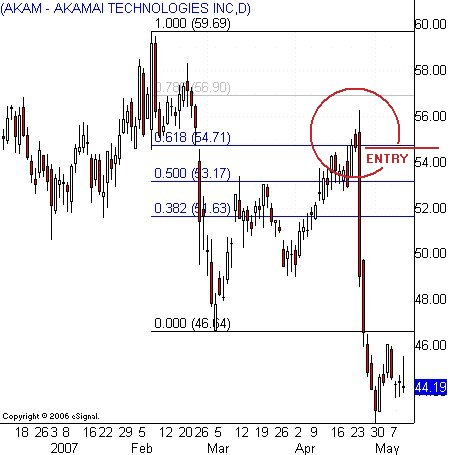# Fibonacci: A Ratio

No comment 608 views
5 / 5 ( 1votes )

Fibonacci: A RatioFibonacci: A Ratio

The Fibonacci series is derived by finding the sum of the two preceding numbers in a series or series, with 0 and 1 being the core number, or starting point. Figures 0 and 1 become the first two core numbers; the third number in the series is the sum of 0 and 1 (0 + 1), ie 1; the fourth number in the series is the number of the second and third numbers in the series, ie 1 + 1, ie 2, and so on.

As the number of series increases to infinity, mathematical relationships, expressed as ratios, appear among the numbers. For example, the ratio between consecutive numbers tends to be at an approximate 1.618034, known as the golden or golden or golden mean ratio and is denoted by the capitalized Greek letter Phi Φ in mathematics. This ratio is achieved by dividing the number in the series of sum with the preceding number: 233 ÷ 144 = 1.618055.

The opposite of this ratio is 0.618034, which is denoted by the lower case Greek letters phi φ in mathematics. This ratio is achieved by dividing the number in the summation series by the number that follows it: 144 ÷ 233 = 0.618025.

The square of 0.618034 approaches the 0.381966 ratio and when we divide a number in the series of sums by the sum of two numbers thereafter we tend to approach this ratio. We also achieved this ratio by subtracting 0.618034 from 1; 0.618034 x 0.618034 = 0.381966 or 144 ÷ 377 = 0.381962 or 1 – 0.618034 = 0.381966. Miraculously huh?

If we multiply the ratio 0.381966 with 0.618034, we reach the ratio of 0.236068 and when we divide the numbers in the sum series by the number of three numbers after that we tend to approach this ratio. We also achieve this ratio when we reduce the ratio 0.381966 from 0.618034; 0.381966 x 0.618034 = 0.236068 or 144 ÷ 610 = 0.236065 or 0.31966 – 0.618034 = 0.236068.

The square root of 0.618034 gives us an approximate ratio of 0.786152: √0.618034 = 0.786152.

The square root of 1.618034 gives us an approximate ratio of 1.272020: √1.618034 = 1.272020.

The square of 1.618034 approaches the ratio 2.168034 and when we divide a number in the series of sums by the sum of the previous two numbers we tend to approach this ratio: 1.618034 x 1.618034 = 2.618034 or 144 ÷ 55 = 2.618181.

When we multiply 2.618034 with 1.618034 we reach the ratio 4.236068 and when we divide a number in the series of sums by the number of the previous three numbers we tend to approach this ratio: 2.618034 x 1.618034 = 4.236068 or 144 ÷ 34 = 4.235294.

This ratio, along with a 50.0%, 100% ratio, forms the key Fibonacci levels used in the technical chart study: 23.6%, 38.2%, 50.0%, 61.8%, 78.6 %, 100.0%, 127.2%, 161.8%, 216.8%, 423.6%. Of these levels, 38.2%, 61.8% and 161.8% were the most significant.Forex Signal 30 is the best forex system since 2009 and has been used by thousands of traders from around the world to generate profit in forex trading. This system is created by our team of Brilliant Forex Signal Team, this system is made as simple as possible for beginner and professional traders.